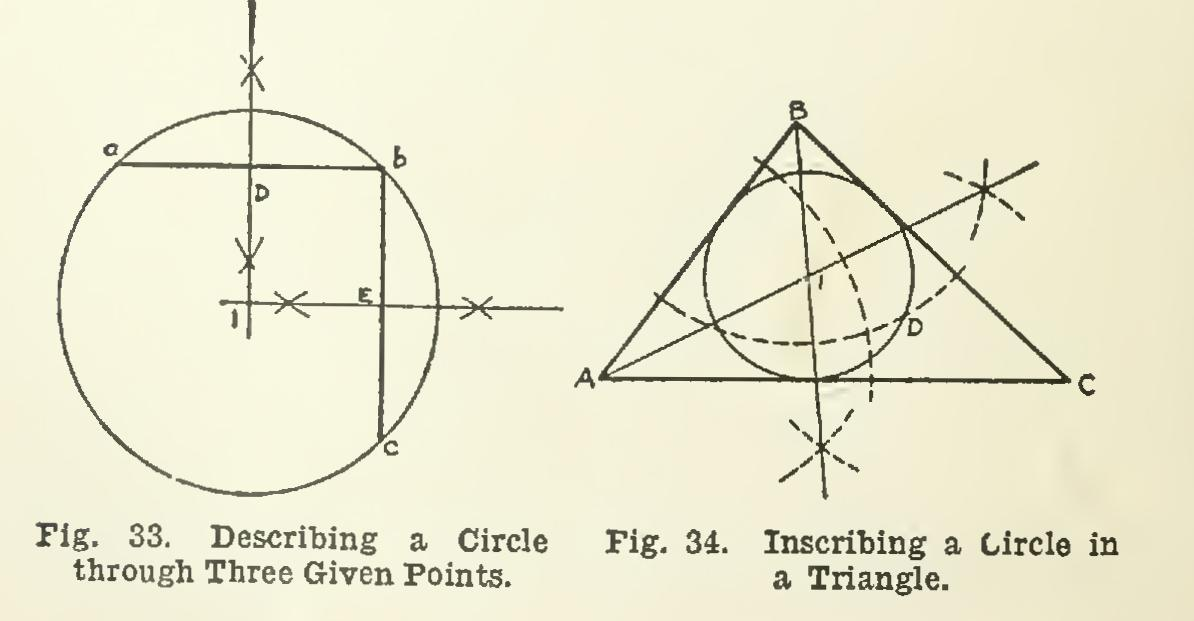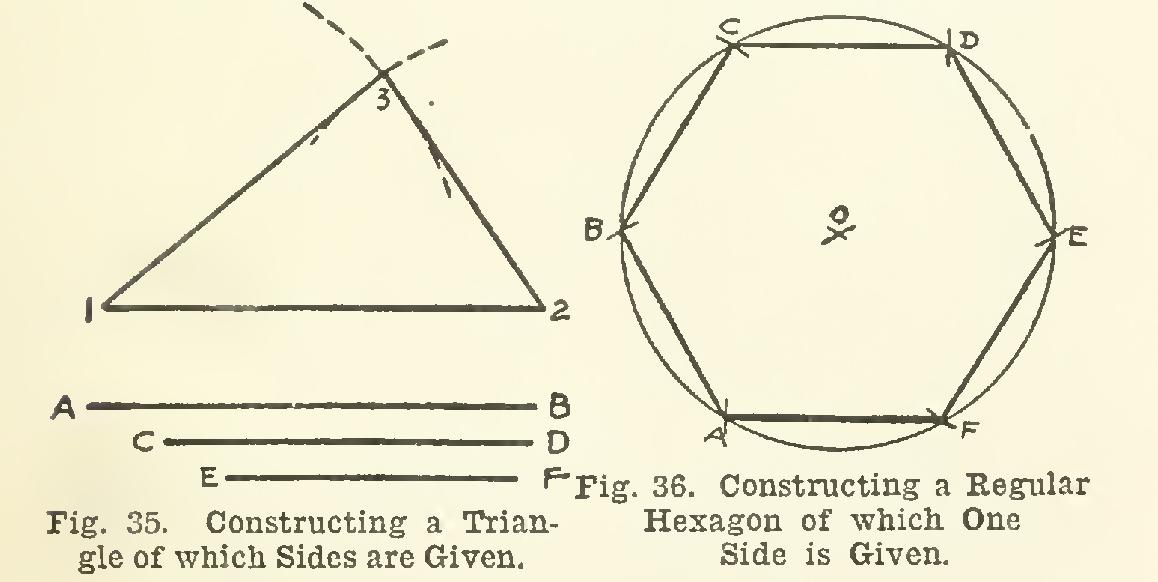Second

line, draw, center, radius, arc, ab and equal

SECOND CASEŚWhen the point on the given line is at or near one end.

Let AB, Fig. 29, be the given line, and c the given point. With point c as center, and any radius, draw an arc cutting AB at point 1; then, with 1 as a center, and the same radius, strike an arc cutting the first one at point 2; and with 2 as center, and the same radius, draw a third arc, cutting the first arc again at point 3. Now, with 2 and 3 in turn as centers, and any con venient radius, strike two arcs intersecting at point 4. A line joining 4 and c is the required perpendicular.

To Draw a Line Parallel to a Given Line and at a Definite Distance Away In Fig. 30, let AB be the given line, and one inch the required distance away for the desired line. With any two points on the line AB, as 1 and 2, and the distance one inch as radius, describe two arcs, as C and D; and with the edge of the triangle, draw a line EF just touch ing or just tangent to these two arcs, and this will be the required line.

-The greater the distance of one line from the other, the less accurate is this construction. For a con siderable distance between the two lines, an accurate construction would be to erect perpendiculars at points 1 and 2 by the method of Problem 5, mark points on these perpendiculars at the required distanec from AB, and draw the required line through these points.

To Divide a Given Straight Line into Any Desired Number of Equal Parts Let AB, Fig. 31, be the given line to be divided into, say, five equal parts. This con struction is, of course, in case the division can not be exactly made with the scale. From either end of the line, as A, draw a straight line AX of indefinite length, at any convenient angle; and lay off on AX, beginning at A, any five equal spaces, A-1, 1-2, 2-3, 3-4, and 4-5. Join point 5 with B; and from points 4, 3, 2, and 1, draw by means of the triangles (Article 28) parallels to 5-B, intersecting AB in points F, E, D, and C, and these points will divide AB into five equal spaces.

To Construct One Angle Equal to Another In Fig. 32, let the angle ABC be the given one, and let it be required to construct on DE as one side an angle equal to ABC. With B as center, and any radius, describe an arc cut ting AB and BC at points 1 and 2; and with D as center, and the same radius, strike a second arc F meeting DE at point 3. Then take 2 as

center, and draw a short arc through point 1; and with this radius, and 3 as center, draw a short arc intersecting arc 3F at 4. Then a line connecting 4 and D will form with the line DE an angle equal to ABC.To Draw a Circle through Three Points Not in the Same Straight Line In Fig. 33, a, b, and c are the given points. Connect a with b, and b with c; and then, by the method of Problem 1, bisect each line. These bisectors D and E intersect at a point 1, which is equally distant from a, b, and c. Hence this point is the center of a circle which will pass through the three points.As three points not in the same straight line may always be connected to form a triangle, it follows that this construction may be used for drawing a circle through the three corners of a triangle, or as it is called, circumscribing a circle about a triangle.

To Inscribe a Circle in a Given Triangle Let ABC, Fig. 34, be the given triangle. By the method of Problem 2, bisect any two of the angles of the triangle, as the angle at A and the one at B; the bisecting lines will intersect each other at point 1. This point is equally distant from all three sides of the triangle; hence, with 1 as center, the required circle D is drawn just tangent to the sides of the tri angle, and is the required inscribed circle.To Construct a Triangle, Having Given the Lengths of the Sides In Fig. 35, let AB, CD, and EF be the lengths of the sides. Draw line 1-2, and make it equal to AB. Then, with point 1 as center, and a radius equal to one of the other sides, as CD, strike an arc; and with point 2 as center, and a radius equal to the remaining side, EF, draw another arc, intersecting the first one at point 3. Join 3 with 1 and 2, and the triangle is com pleted.

To Construct a Regular Hexagon, Having Given One Side In Fig. 36, AP is the given side. With points A and F as centers, and a radius each time equal to length AF, strike arcs intersecting in point 0; and with o as center, and the same radius, draw a circle through A and F. Then, with A as center, and the same radius, draw a short arc cutting the circle at B; then, with center B, radius unchanged, cut the circle again at C; repeat this process, beginning with F, obtain ing points E and D. Draw AB, BC, CD, DE, and EF, and the hexagon is constructed.